# 2nd PUC Maths Question Bank Chapter 8 Application of Integrals

Students can Download 2nd PUC Maths Chapter 8 Application of Integrals Questions and Answers, Notes Pdf, 2nd PUC Maths Question Bank with Answers helps you to revise the complete Karnataka State Board Syllabus and to clear all their doubts, score well in final exams.

## Karnataka 2nd PUC Maths Question Bank Chapter 8 Application of Integrals

### 2nd PUC Maths Application of Integrals Three Marks Questions and Answers

Question 1.
Find the area of the region bounded by the curve y2 = x and the lines x = 4, x = 9 and the x – axis in the first quadrant.
Here a = 4, b = 9 and y = √x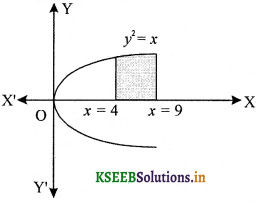Question 2.
Find the area of the region bounded by the curve y2 = x and the lines x = 1, x = 4 and the X-axis.
The area of the region bounded by the curve, y2 = x, the lines x = 1 and x = 4 and the X-axis is shown in the figure.Here a = 1, b = 4
Since, the given curve y2 = x is parabola which is symmetrical about X-axis (∵ it contains even power of y) and passes through the origin.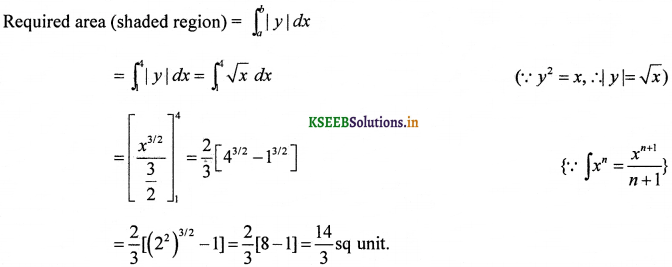Question 3.
Find the area of the region bounded by y2 = 9x, x = 2, x = 4 and the X-axis in the first quadrant.
Since, the given curve y2 = 9x is a parabola which is symmetrical about X-axis (∵ the power ofy is even) and passes through the origin.
The area of the region bounded by the curve, y2 = 9x, x = 2 and x = 4 and the X-axis is the area shown in the figure.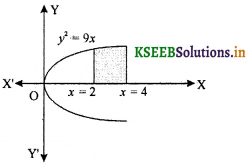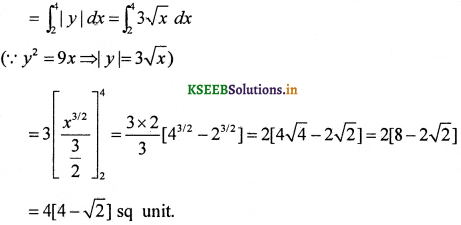Question 4.
Find the area of the region bounded by x2 = 4y, y = 2, y = 4 and the Y-axis in the first quadrant.
The given curve x2 = 4y is a parabola which is symmetrical about Y-axis (∵ it contains even power of x) only and passes through the origin.
The area of the region bounded by the curve x2 = 4y, y = 2 and y = 4 and the Y-axis is shown in the figure.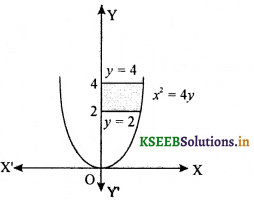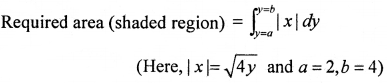Question 5.
Find the area of the region bounded by the parabola y = x2 and y = |x|.
Given parabola y = x2 which is symmetrical about Y-axis and passes through (0, 0) and the curve y = |x|.
On putting x = – x, we gety = |-x| = |x|
∴ Curve y = |x| is symmetrical about Y-axis and passes through origin.
The area bounded by the parabola, y = x2 and the line y = |x| or can be represented in the figure.The point of intersection of parabola, and line, y = x in first quadrant is A (1, 1).
∴ Area OACO = Area ODBO
Required area = 2 (Area of shaded region in the first quadrant only)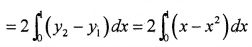(∵ The curve y = |x| lies above the curve y = x2 in [0, 1] so, we take y2 = x and y1 = x2)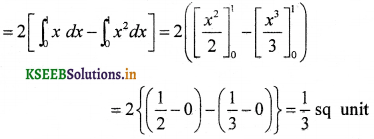Therefore, required area is $$\frac { 1 }{ 3 }$$ sq unit.Question 6.
Find the area of the region bounded by the curve y2 = 4x and the line x = 3.
The given curve is y2 = 4x, which represents a right hand parabola with vertex. at (0, 0) and axis along X-axis and the equation of line is x = 3.
Required area = 2 × (Area of shaded region in the first quadrant only)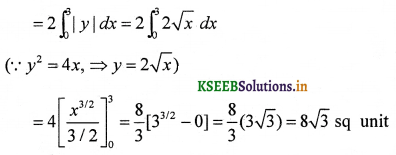Therefore, required area is 8√3 sq unit.

Question 7.
Find area lying between the curves y2 = 4x and y = 2x is
The given curve is y2 = 4x ……. (i)
and the given lineis y = 2x ……… (ii)
The two curve meet where (2x)2 = 4x ⇒ 4x(x – 1) = 0 ⇒ x = 0, 1
When x = 0, y = 2 × 0 = 0 and when x = 1, y = 2 × 1 = 2
∴ Eqs. (i) and (ii) meet at the points (0, 0) and (1, 2).Question 8.
Find the area of the region bounded by the curve y = x2 and the line y = 4.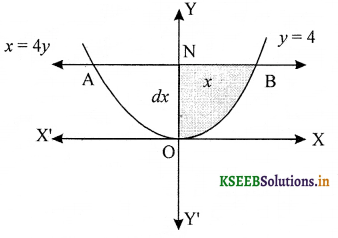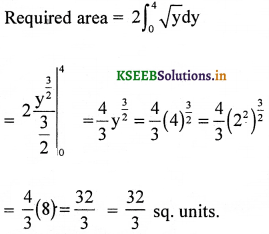### 2nd PUC Maths Application of Integrals Five Marks Questions and Answers

Question 1.
Find the area of the region bounded by the ellipse $$\frac{x^{2}}{16}+\frac{y^{2}}{9}=1$$.
The given curve is an ellipse with centre at (0,0) and symmetrical about X-axis and Y-axis (∵ the power of x and y both are even).
Area bounded by the ellipse = 4 × (Area of shaded region in the first quadrant only)
(∵ By symmetry)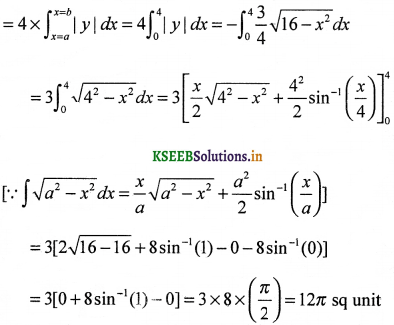Therefore, area bounded by the ellipse is 12 π units.Question 2.
Find the area of the region bounded by the ellipse $$\frac{x^{2}}{4}+\frac{y^{2}}{9}$$ = 1.
The given curve is an ellipse with centre at (0, 0) and symmetrical about X-axis and Y-axis
Area bounded by the ellipse = 4 × (Area of shaded region in the first quadrant only)
(∵ By symmetry)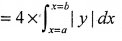Question 3.
Find the area of the region in the first quadrant enclosed by X-axis and x = √3y and the circle x2 + y2 = 4.
Given equation of circle x2 + y2 = 4 and x = √3y or y = $$\frac{1}{\sqrt{3}}$$x represents a line through the origin.
The line y = $$\frac{1}{\sqrt{3}}$$x intersect the circle so it will satisfy the equation of circle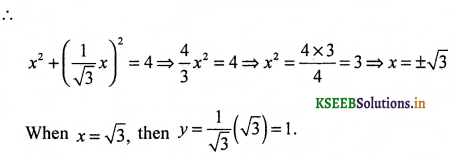When x=J, then
[For first quadrant we take x = √3 and neglect x = -√3]
∴ The line and the circle meet at the point (√3,1).
= (Area under the line y = $$\frac{1}{\sqrt{3}}$$x from x = 0 to x = √3)
+ (Area under the circle from x = √3 to x = 2)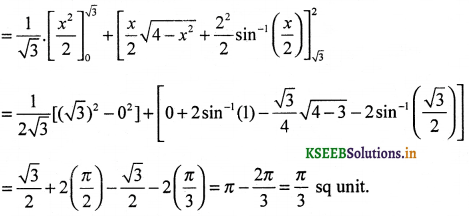Question 4.
Find the area of the smaller part of the circle x2 + y2 = a2 cut-off by the line x = $$\frac{a}{\sqrt{2}}$$
Given line, x = $$\frac{a}{\sqrt{2}}$$
and circle, x2 + y2 = a2
Since, given line cuts the circle so put x = $$\frac{a}{\sqrt{2}}$$
Eq. (ii), we get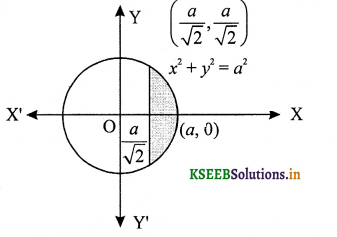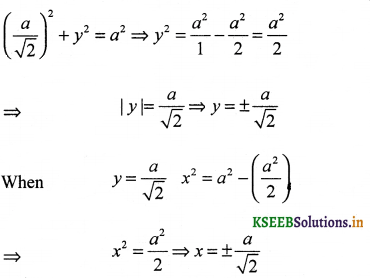∴ Intersection point in first quadrant is $$\left(\frac{a}{\sqrt{2}}, \frac{a}{\sqrt{2}}\right)$$
Required area = 2 (Area of shaded region in first quadrant only)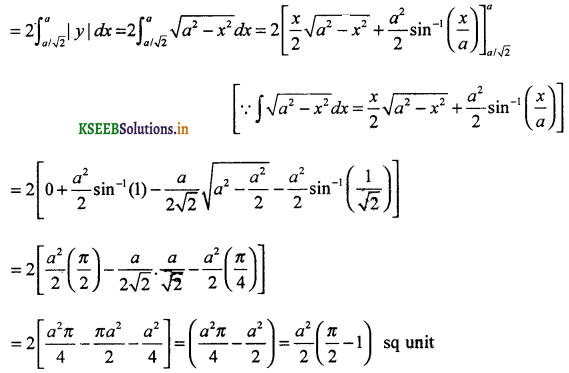Therefore, the area of smaller part of the circle, x2 + y2 = a2, cut-off by the line,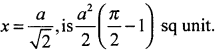Question 5.
The are between x = y2 and x = 4 is divided into two equal parts by the line x = a, find the value of a.
Given curve x = y2 is a parabola symmetrical about X-axis and passing through the Origin.
The line x = a, divides the area bounded by the parabola and x = 4 into two equal parts.
∴ Area OED = Area EFCD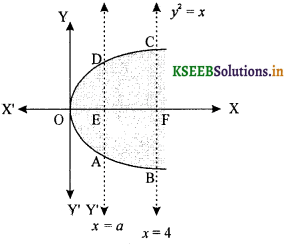⇒ Area OED = $$\int_{0}^{a} y d x$$
and area of EFCD = $$\int_{a}^{4} \sqrt{x} d x$$
(∵ y2 = x ⇒ |y| = √x)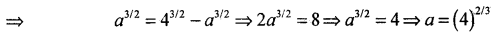Therefore, the value of a is (4)2/3

Question 6.
Find the area bounded by the curve x2 = 4y and the line x = 4y – 2.
Given curve is x2 = 4y ….. (i)Which represents an upward parabola with vertex (0,0) and axis along Y-axis and the equation of strainght line x = 4y – 2 …..(ii)
For intersection point put the value of 4y from Eq. (i) in Eq. (ii), we get
x2 = x + 2
⇒ x2 – x – 2 = 0
⇒ (x – 2) (x + 1) = 0
⇒ x = 2, – 1
When x = 2, then from Eq. (ii), we get
4y = 2 + 2
⇒ y = 1
When x = – 1, then from Eq.
(ii), we get 4y = 2 – 1 = 1 ⇒ y = $$\frac { 1 }{ 4 }$$
∴ The line meets the parabola at the points B$$\left(-1, \frac{1}{4}\right)$$ and A(2, 1).
Required area = (Area under the line x = 4y – 2) – (Area under the parabola x2 = 4y)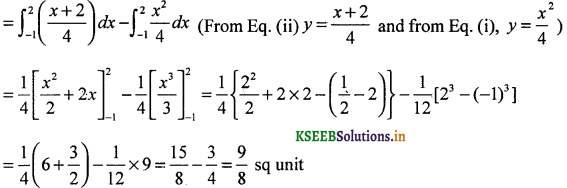Therefore, required area is $$\frac { 9 }{ 8 }$$ sq unit.Question 7.
Find the area of the circle 4x2 + 4y2 = 9 which is interior to the parabola x2 = 4y.
Given, circle is 4x2 + 4y2 = 9 and the given parabola is x2 = 4y. The two curves meet where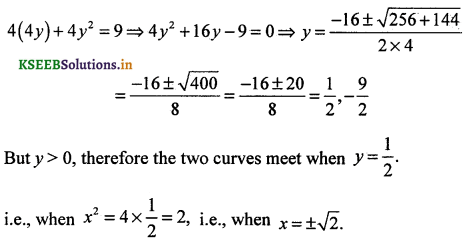∴ Required area (shown in shaded region)Question 8.
Find the area bounded by the curves (x – 1)2 + y2 = 1 and x2 + y2 = 1.
(x – 1)2 + y2 = 1 ……. (i)
∴ y = $$\sqrt{1-(x-1)^{2}}$$
which represents a circle with centre (1, 0) and radius 1
and curve x2 + y2 = 1 ……. (i)
∴ y = $$\sqrt{1-x^{2}}$$
which represents a circle with centre (0, 0) and radius 1
Both the curves are circle and meet where (x – 1)2 = x2 i.e., where 2x = 1 or x = $$\frac { 1 }{ 2 }$$
Required area (shown in shaded region).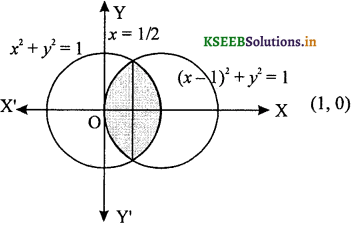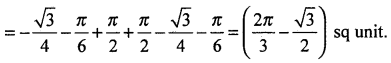Question 9.
Find the area of the region bounded by the urves y = x2 + 2, y = x, x = 0 and x = 3.
Given curve y = x2 + 2
represents a parabola and it is symmetrical about Y-axis having vertex (0,2).
The given region bounded by y = x2 + 2, y = x, x = 0 and x = 3, is represented by the shaded area.
The point of intersection of the curve y = x2 + 2 and the line x = 3 is (3, 11).
Required area (shown in shaded region)
= Area OABDO – Area OCDO = [Area under y = x2 + 2 between x = 0, x = 3] – [Area under y = x between y = x between x = 0, x = 3]Question 10.
Using integration, find the area of region bounded by the triangle whose vertices are (-1, 0), (1, 3) and (3, 2).
Let the given points are A(-1, 0), B(1, 3) and C(3,2).
Equation of the line joing points (x1, y1) and (x2, y2) is
(y – y1 = $$\frac{y_{2}-y_{1}}{x_{2}-x_{1}}$$(x – x1)
Equation of line AB is y – 0 = $$\frac{3-0}{1+1}$$ (x + 1) ⇒ y = $$\frac{3}{2}$$ (x + 1)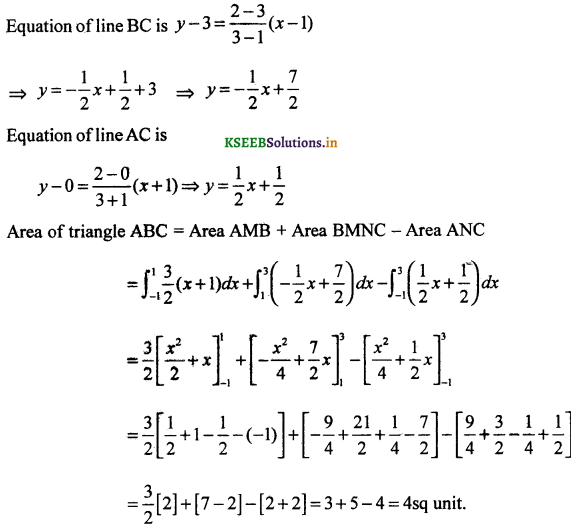Question 11.
Using integration, find the area of the triangular region whose sides have the equations
y = 2x + 1, y = 3x + 1 and x = 4.
Given equation of sides of the triangle are y = 2x + 1, y = 3x + 1 and x = 4.
On solving these equations, we obtain the vertices of triangle as A(0, 1), B(4, 13) and C(4, 9).
∴ Required area (shown in shaded region) :
Area (OLBAO) – Area (OLCAO)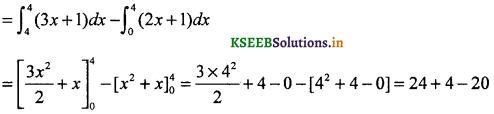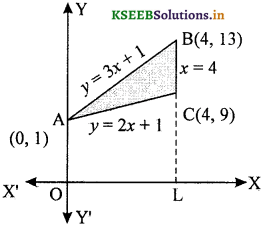Question 12.
Find the smaller area enclosed by the circle x2 + y2 = 4 and the line x + y = 2
The smaller area enclosed by the circle, x2 + y2 = 4 and the line x + y = 2 is represented by the shaded area ACBA.
The intersection points of circle and line are A(2,0) and B(0,2).
∴ Required area (shown in shaded region)
= Area OACBO – Area (∆ OAB)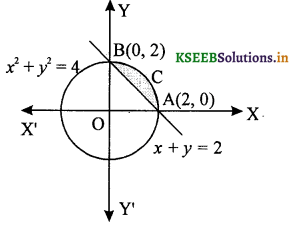Question 13.
find the area of the region enclosed by the parabola x2 = y, the Iiney = x + 2 and the X-axis.
given the curve (represent an up ward parabola with vertex (0, 0))
y = x2 …. (i)
and equation of the line y = x + 2 ….. (ii)
The line and parabola meet where x2 = x + 2 (Eliminating y)
∴ x2 – 2 = 0 ⇒ (x – 2)(x + 1) = 0 ⇒ x = 2 or -1
When x = 2, y = 2 + 2 = 4
When x = -1, y = (-1) + 2 = 1
Thus, the point of intersection of the parabola and line are (-1, 1) and (2, 4).
Required area = (Area under the line y = x + 2, between x = – 1, x = 2) – (Area under the curve x2 = y, between x = – 1, x = 2)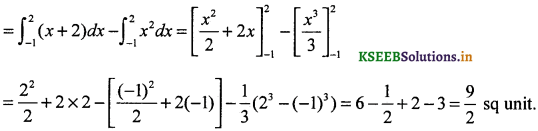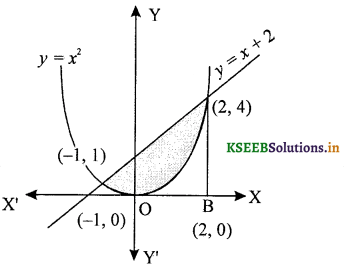Question 14.
Using method of integration find bounded by the curve |x| + |y| = 1.
The given curve is |x| + |y| = 1
In first quadrant, (x > 0, y > 0)
Then, the line AB is x + y = 1
In second quadrant, (x < 0, y > 0)
then, the line BC is – x + y = 1
In third quadrant (x < 0, y < 0)
then, the line CD is – x – y = 1
In fourth quadrant (x > 0, y < 0)
Then, the line DA is x – y = 1
Since, ABCD is a square.
∴ Required area = 4 (Area of shaded region in the first quadrant)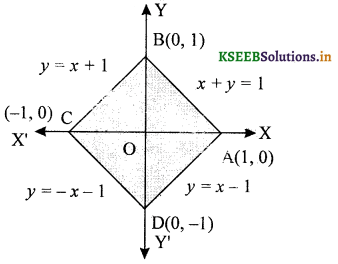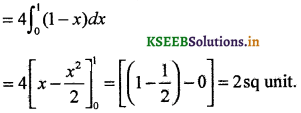Question 15.
Find the area bounded by curves x2 – y and y = |x|
Given the curve y = x2 ……. (i)
and equation of line y = |x|
In first quadrant, the equation of the line is y = x
and in second quadrant, the equation of line is y = – x.
The points of intersection of the parabola and line are (-1, 1) and (1, 1). As the area to be found is symmetrical y = about Y-axis.
∴ Required area = 2 (Area of shaded region in the first (-1, 1) quadrant)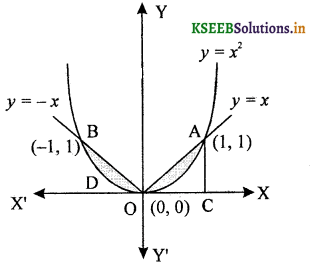Question 16.
Using the method of integration find the area of the triangle ABC, coordinates of whose vertices are A(2, 0), B(4, 5) and C(6, 3).
The given points area A(2,0), B(4,5) and C(6, 3)
Equation of line AB is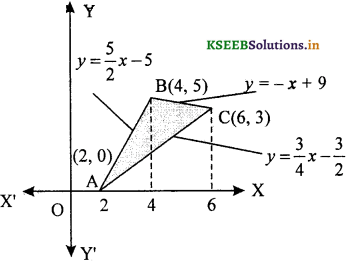Equation of line BC is
y – 5 = $$\frac{3-5}{6-4}$$( x – 4) ⇒ y = -x + 9
Equation of line AC is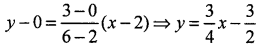Required area = (Area under line segment AB) + (Area under line segment BC) – (Area under line segment AC)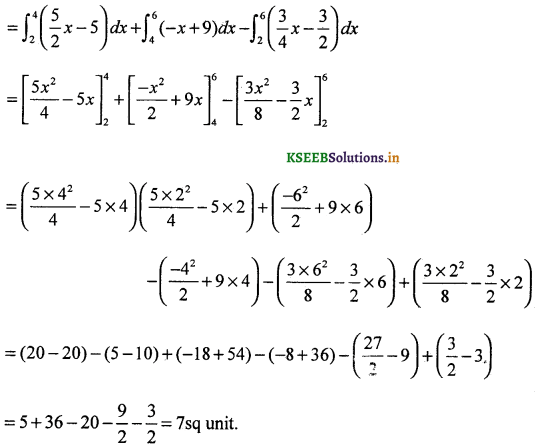Question 17.
Find the area of the region y2 = 4x and 4x2 + 4y2 = 9
given curve y2 = 4x ……. (i)
and circle 4x2 + 4y2 = 9 …. (ii)
Which represents a circle whose centre is (0, 0) and radius $$\frac { 3 }{ 2 }$$
For intersecting points of parabola and circle put the value of y2 from Eq. (i) in Eq. (ii), we get4x2 + 16x = 9
⇒ 4x2 + 16x – 9 = 0
⇒ 4x2 + 18x – 2x – 9 = 0
⇒ 2x (2x + 9) – 1 (2x + 9) = 0
⇒ (2x + 9)(2x – 1) = 0
⇒ x = $$\frac{-9}{2}, \frac{1}{2}$$ ⇒ x = $$\frac{1}{2}$$
(x ≠ $$\frac{-9}{2}$$ because it does not satisfy the given inequality)
On putting x = $$\frac{1}{2}$$ in Eq. (i) we get y = ±√2
∴ The points of intersection of both the curves are A $$\left(\frac{1}{2}, \sqrt{2}\right)$$ and
∴ Required area = 2 (Area OABO)